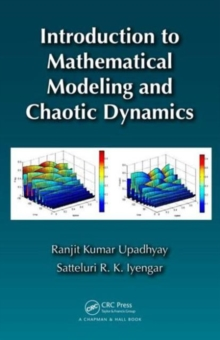Supporting your high street Find out how »
• My Account# Introduction to Mathematical Modeling and Chaotic Dynamics Hardback

#### Description

Introduction to Mathematical Modeling and Chaotic Dynamics focuses on mathematical models in natural systems, particularly ecological systems.

Most of the models presented are solved using MATLAB (R). The book first covers the necessary mathematical preliminaries, including testing of stability.

It then describes the modeling of systems from natural science, focusing on one- and two-dimensional continuous and discrete time models.

Moving on to chaotic dynamics, the authors discuss ways to study chaos, types of chaos, and methods for detecting chaos.

They also explore chaotic dynamics in single and multiple species systems.

The text concludes with a brief discussion on models of mechanical systems and electronic circuits. Suitable for advanced undergraduate and graduate students, this book provides a practical understanding of how the models are used in current natural science and engineering applications.

Along with a variety of exercises and solved examples, the text presents all the fundamental concepts and mathematical skills needed to build models and perform analyses.

#### Information

• Format: Hardback
• Pages: 363 pages
• Publisher: Taylor & Francis Ltd
• Publication Date:
• Category: Mathematical foundations
• ISBN: 9781439898864

#### Other Formats

£77.99

£68.49

on all orders

###### Pick up orders

from local bookshops

£54.99

£48.95